Test: Basic BJT Circuits

# Test: Basic BJT Circuits

Test Description

## 20 Questions MCQ Test GATE Electrical Engineering (EE) 2023 Mock Test Series | Test: Basic BJT Circuits

Test: Basic BJT Circuits for Electronics and Communication Engineering (ECE) 2023 is part of GATE Electrical Engineering (EE) 2023 Mock Test Series preparation. The Test: Basic BJT Circuits questions and answers have been prepared according to the Electronics and Communication Engineering (ECE) exam syllabus.The Test: Basic BJT Circuits MCQs are made for Electronics and Communication Engineering (ECE) 2023 Exam. Find important definitions, questions, notes, meanings, examples, exercises, MCQs and online tests for Test: Basic BJT Circuits below.
Solutions of Test: Basic BJT Circuits questions in English are available as part of our GATE Electrical Engineering (EE) 2023 Mock Test Series for Electronics and Communication Engineering (ECE) & Test: Basic BJT Circuits solutions in Hindi for GATE Electrical Engineering (EE) 2023 Mock Test Series course. Download more important topics, notes, lectures and mock test series for Electronics and Communication Engineering (ECE) Exam by signing up for free. Attempt Test: Basic BJT Circuits | 20 questions in 60 minutes | Mock test for Electronics and Communication Engineering (ECE) preparation | Free important questions MCQ to study GATE Electrical Engineering (EE) 2023 Mock Test Series for Electronics and Communication Engineering (ECE) Exam | Download free PDF with solutions
 1 Crore+ students have signed up on EduRev. Have you?
Test: Basic BJT Circuits - Question 1

### In a Darlington pair configuration, if emitter resistance is 560Ω and input resistance is 8.75MΩ than AC current gain will be

Test: Basic BJT Circuits - Question 2

### For the transistor in circuit shown in fig. β = 200. Determine the value of VC for given value of VB in question.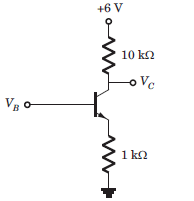If VB = 1V

Detailed Solution for Test: Basic BJT Circuits - Question 2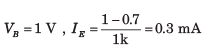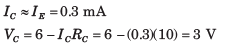Test: Basic BJT Circuits - Question 3

### For the transistor in circuit shown in fig. β = 200. Determine the value of IE and IC for given value of VB in question.If VB = 2V

Detailed Solution for Test: Basic BJT Circuits - Question 3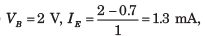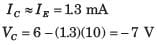Transistor is in stauration. The saturation voltage
VCE = 0.2V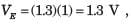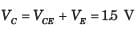Test: Basic BJT Circuits - Question 4

The transistor in circuit shown in fig. has β = 200. Determine the value of voltage Vo for given value of VBB.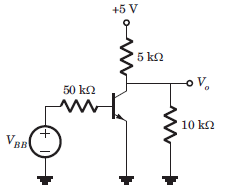If VBB = 0

Detailed Solution for Test: Basic BJT Circuits - Question 4

VBB = 0, Transistor is in cutoff region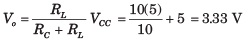Test: Basic BJT Circuits - Question 5

The transistor in circuit shown in fig. has β = 200. Determine the value of voltage Vo for given value of VBB.If VBB = 1V

Detailed Solution for Test: Basic BJT Circuits - Question 5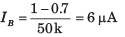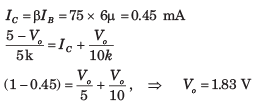Test: Basic BJT Circuits - Question 6

The transistor in circuit shown in fig. has β = 200. Determine the value of voltage Vo for given value of VBB.If VBB = 2V

Detailed Solution for Test: Basic BJT Circuits - Question 6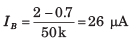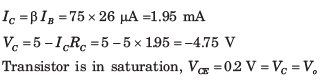Test: Basic BJT Circuits - Question 7

The transistor shown in the circuit of fig. has β = 150. Determine Vo for given value of
IQ in question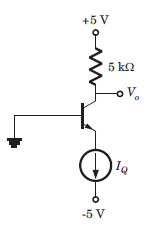If IQ = 0.1 mA

Detailed Solution for Test: Basic BJT Circuits - Question 7

I= 0.1 mA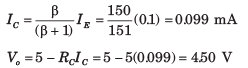Test: Basic BJT Circuits - Question 8

The transistor shown in the circuit of fig. has β = 150. Determine Vo for given value of  IQ in questionIf IQ = 0.5 mA

Detailed Solution for Test: Basic BJT Circuits - Question 8

IE = IQ = 0.5 mA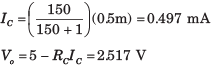Test: Basic BJT Circuits - Question 9

The transistor shown in the circuit of fig. has β = 150. Determine Vo for given value of  IQ in questionIf IQ = 2mA

Detailed Solution for Test: Basic BJT Circuits - Question 9

Transistor is in saturation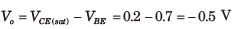Test: Basic BJT Circuits - Question 10

For the circuit in fig. VB = V and  β = 50. The value of VB is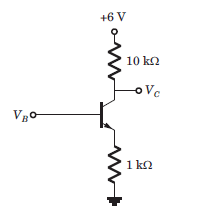Detailed Solution for Test: Basic BJT Circuits - Question 10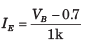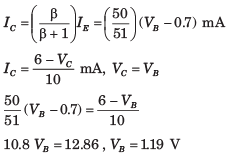Test: Basic BJT Circuits - Question 11

For the circuit shown in fig. VCB = 0.5 V and β =100. The value of IQ is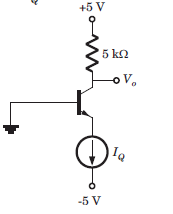Detailed Solution for Test: Basic BJT Circuits - Question 11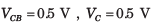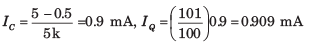Test: Basic BJT Circuits - Question 12

For the circuit shown in fig. the emitter voltage is VE = 2 V. The value of α is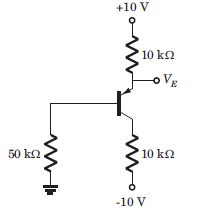Detailed Solution for Test: Basic BJT Circuits - Question 12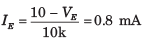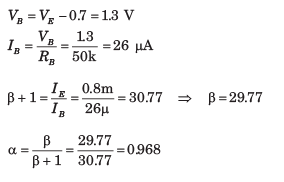Test: Basic BJT Circuits - Question 13

For the transistor in fig. β = 50. The value of voltage VEC  is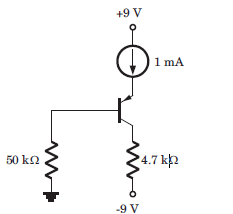Detailed Solution for Test: Basic BJT Circuits - Question 13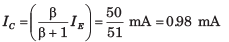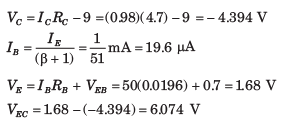Test: Basic BJT Circuits - Question 14

The current gain of the transistor shown in the circuit of fig. is β = 125. The Q-point values (ICQ , VCEQ ) are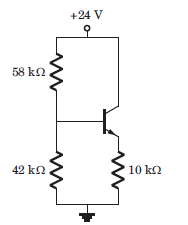Detailed Solution for Test: Basic BJT Circuits - Question 14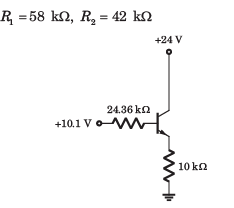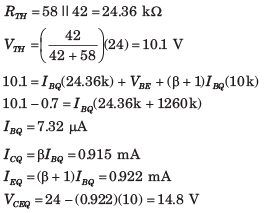Test: Basic BJT Circuits - Question 15

For the circuit shown in fig. , let β = 75. The Q-point (ICQ , VCEQ ) is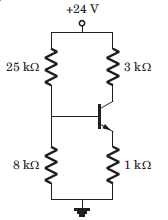Detailed Solution for Test: Basic BJT Circuits - Question 15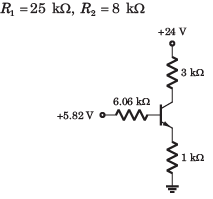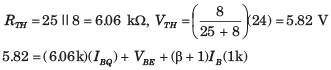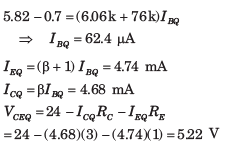Test: Basic BJT Circuits - Question 16

The current gain of the transistor shown in the circuit of fig. is β = 100. The values of Q-point (ICQ , VCEQ ) is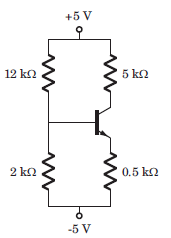Detailed Solution for Test: Basic BJT Circuits - Question 16

R1 =12 Ω, R2 = 2 Ω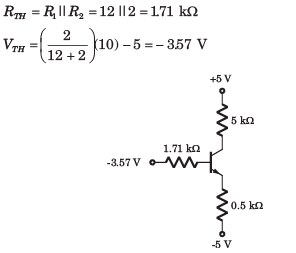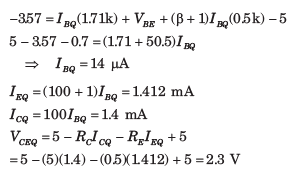Test: Basic BJT Circuits - Question 17

For the circuit in fig. , let β = 60. The value of VECQ  is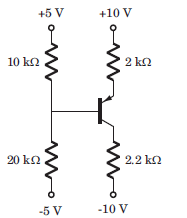Detailed Solution for Test: Basic BJT Circuits - Question 17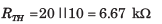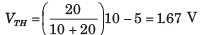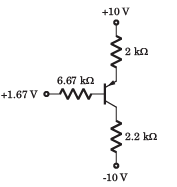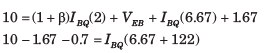Test: Basic BJT Circuits - Question 18

All transistor in the N output mirror in fig. are matched with a finite gain β and early voltage VA = ∞. The expression for each load current is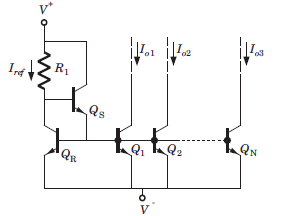Detailed Solution for Test: Basic BJT Circuits - Question 18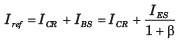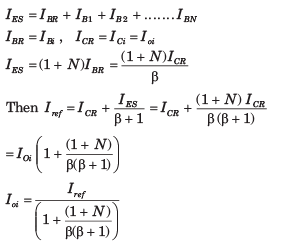Test: Basic BJT Circuits - Question 19

Consider the basic three transistor current source in fig. Assume all transistor are matched with finite gain and early voltage VA  = ∞.The expression for Io is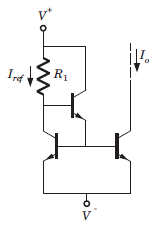Detailed Solution for Test: Basic BJT Circuits - Question 19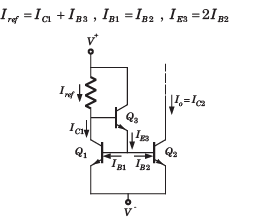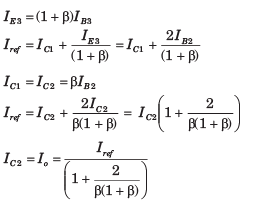Test: Basic BJT Circuits - Question 20

Consider the wilder current source of fig. Both of transistor are identical and β>>1 and VBE1 = 0.7 V. The value of resistance R1 and RE to produce Iref  = 1 mA and Io = 12 μA is (Vt = 0.026)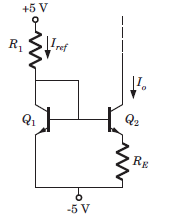Detailed Solution for Test: Basic BJT Circuits - Question 20

If β>>1 and transistor are identical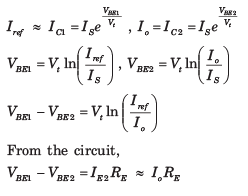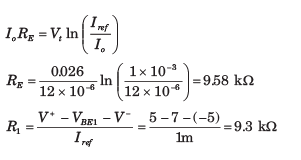## GATE Electrical Engineering (EE) 2023 Mock Test Series

22 docs|274 tests
 Use Code STAYHOME200 and get INR 200 additional OFF Use Coupon Code
Information about Test: Basic BJT Circuits Page
In this test you can find the Exam questions for Test: Basic BJT Circuits solved & explained in the simplest way possible. Besides giving Questions and answers for Test: Basic BJT Circuits, EduRev gives you an ample number of Online tests for practice

## GATE Electrical Engineering (EE) 2023 Mock Test Series

22 docs|274 tests